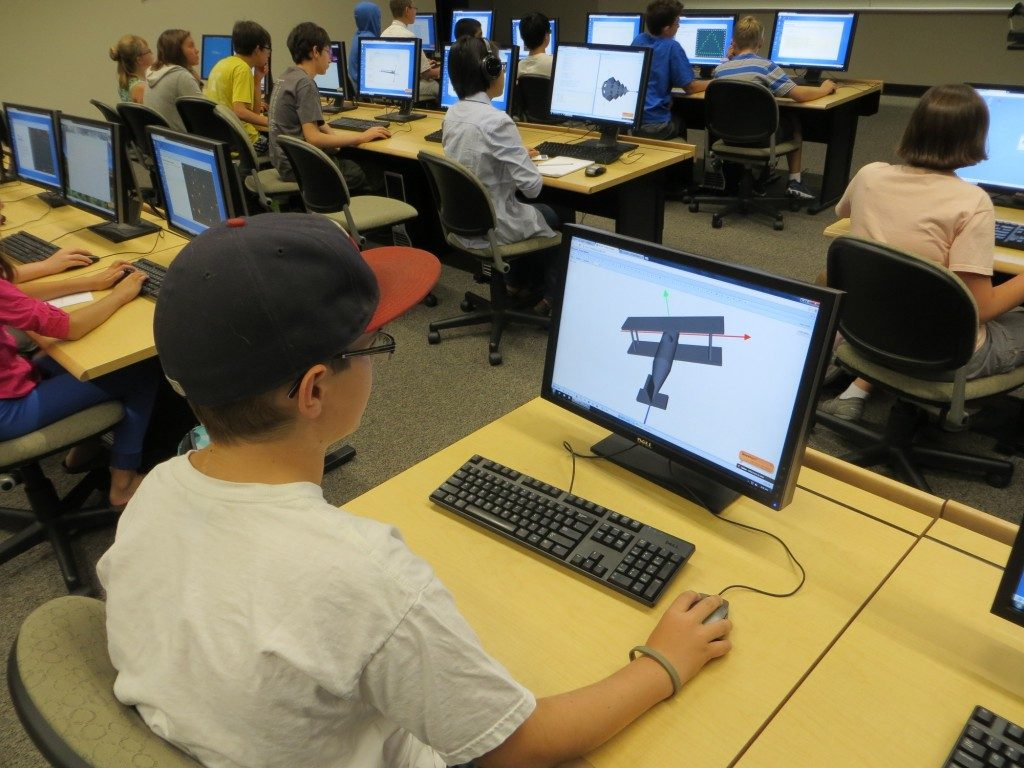# 3D #4: Model with Mathematics

## What It Means

Use math to solve real-world problems, organize data, and understand the world around you.

## 3D Modeling Teaches Students to Understand the Real World

Alex wants to build a 3D model of a biplane and print it on a 3D printer. In order to do this, he needs to learn a lot:

• Variety of geometrical shapes such as boxes, cylinders, cones, and spheres.
• Geometrical transformations including translations, rotations, and scaling.
• Set operations including intersections, unions, and set differences.

Alex also learns that the 3D shape of the model is just a small part of the larger picture. 3D models are further used for engineering analysis to calculate mechanical, thermal, electromagnetic, and other properties of 3D objects. This is called Finite Element Analysis (FEA). NCLab has a FEA module where students can connect 3D modeling with other areas of engineering analysis.